Horizontal scales of convection cells (2) -Estimates of convective velocities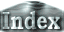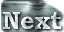Considering the convection development scenario outlined in the previous section further, we can evaluate the convective velocities induced by the vertical and horizontal temperature differences, and compare their amplitudes.

The convective velocity induced by the vertical temperature difference u is obtained from ordinary boundary-layer theory: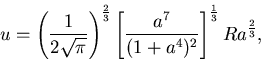(10)
The velocity of convection beneath the plate u' is estimated by substituting for Ra in (10) the value of Ra' in (8). In light of the comparison above with the numerical calculations, we divide equation (10) by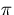to estimate the velocity amplitudes.

Conversely, the velocity amplitude of the convection induced by the horizontal temperature difference uh is determined by the dynamical balance of the equation of motion at steady state: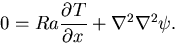(11)
Differentiating (11) with respect to z and using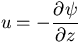, we obtain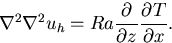(12)
Temperature difference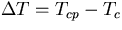, is expressed as a function of the Rayleigh number using (5) and (9). The vertical scale of a convection cell is Lz=1 and the horizontal scale is Lx. Then we have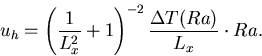(13)
We divide this equation by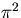to evaluate the velocity amplitude, considering that the distributions of the temperature and stream function are approximately described by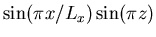.

 Horizontal scales of convection cells (2) -Estimates of convective velocities